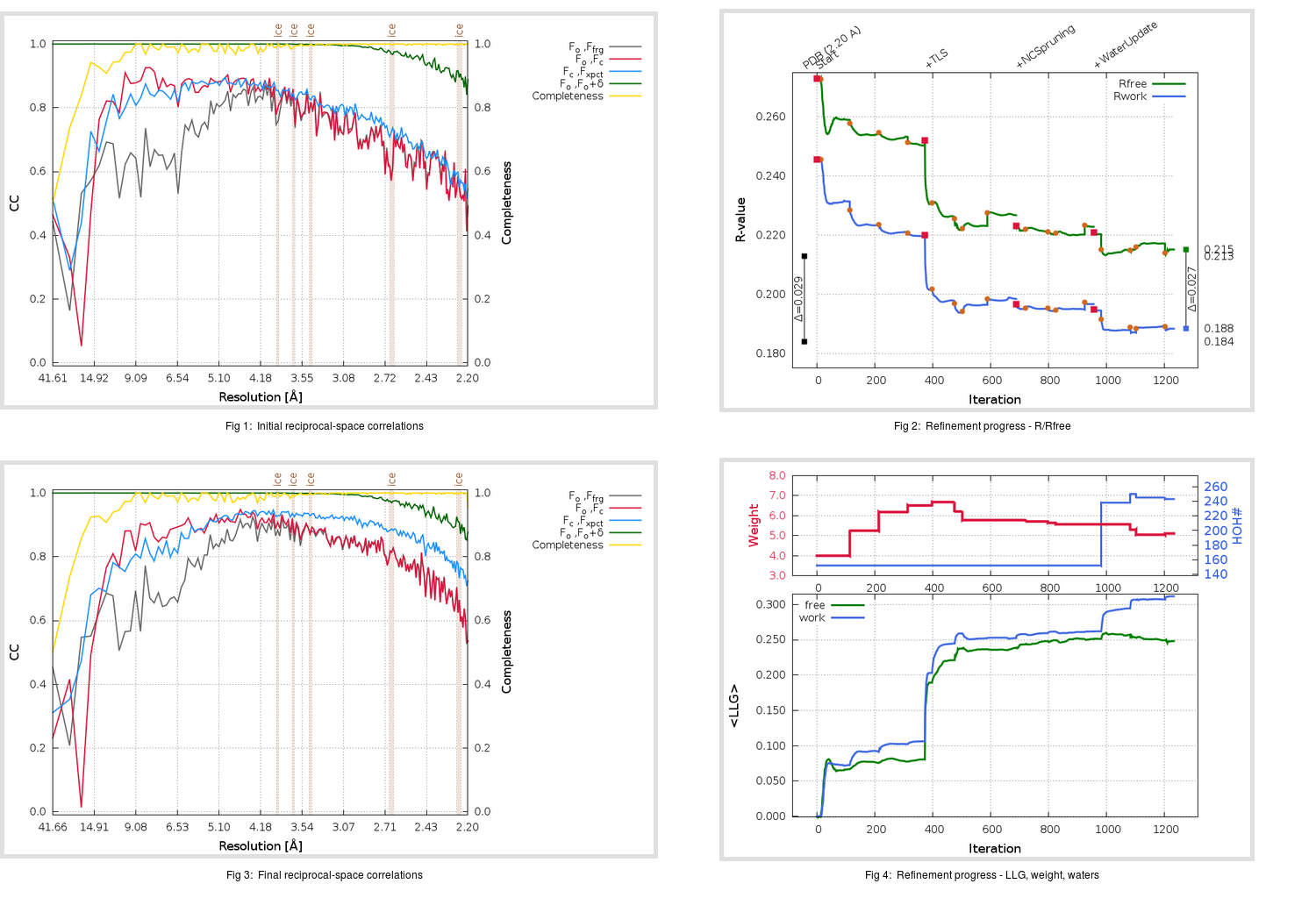Content:

```    Diffraction limits & principal axes of ellipsoid fitted to diffraction cut-off surface:
2.322         1.0000   0.0000   0.0000       a*
2.322         0.0000   1.0000   0.0000       b*
2.084         0.0000   0.0000   1.0000       c*
```

## Deposited

` `
 Date deposited Date data collection Resolution R, Rfree 20060327 20050909 2.20 0.1820 0.2130

Molprobity (CCP4 7.0 version) summary:

```Ramachandran outliers =   0.55 %
favored =  94.75 %
Rotamer outliers      =   6.21 %
C-beta deviations     =     0
Clashscore            =   3.66
RMS(bonds)            =   0.0130
RMS(angles)           =   1.47
MolProbity score      =   2.12
Resolution            =   2.20
R-work                =   0.1820
R-free                =   0.2130
```

```Number of waters      =   152

<B> (all atoms) =   54.55 ( sd =    7.46 ) for       3096 non-hydrogen atoms
<B>   (protein) =   54.60 ( sd =    7.26 ) for       2944 non-hydrogen atoms
<B>     (water) =   53.73 ( sd =   10.55 ) for        152 non-hydrogen atoms
<B>    (others) =    0.00 ( sd =    0.00 ) for          0 non-hydrogen atoms

B min/max       (all non-hydrogen atoms) =   35.63 /   90.95
B min/max   (protein non-hydrogen atoms) =   36.79 /   90.95
B min/max     (water non-hydrogen atoms) =   35.63 /   89.29
B min/max     (other non-hydrogen atoms) =    0.00 /    0.00
```

## BUSTER (re-)refinement

` `

Molprobity (CCP4 7.0 version) summary:

```Ramachandran outliers =   0.55 %
favored =  94.75 %
Rotamer outliers      =   4.97 %
C-beta deviations     =     2
Clashscore            =   2.97
RMS(bonds)            =   0.0117
RMS(angles)           =   1.62
MolProbity score      =   1.98
Resolution            =   2.20
R-work                =   0.1884
R-free                =   0.2152
```

```Number of waters      =   243

<B> (all atoms) =   59.06 ( sd =   18.57 ) for       3187 non-hydrogen atoms
<B>   (protein) =   58.58 ( sd =   18.72 ) for       2944 non-hydrogen atoms
<B>     (water) =   64.86 ( sd =   15.52 ) for        243 non-hydrogen atoms
<B>    (others) =    0.00 ( sd =    0.00 ) for          0 non-hydrogen atoms

B min/max       (all non-hydrogen atoms) =   30.66 /  155.92
B min/max   (protein non-hydrogen atoms) =   30.66 /  147.91
B min/max     (water non-hydrogen atoms) =   35.02 /  155.92
B min/max     (other non-hydrogen atoms) =    0.00 /    0.00
```

Refinement progression:Results:

` `
 File Remark 2GHV_aB_refine.01_04_refine.pdb.gz exact refinement commands are in header 2GHV_aB_refine.01_04_refine.mtz.gz including original deposited data and several re-refinement map coefficients 2GHV_aB_refine.01_04_BUSTER_model.cif.gz including any non-standard compound restraints 2GHV_aB_refine.01_04_BUSTER_refln.cif.gz